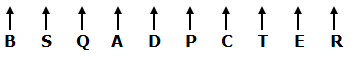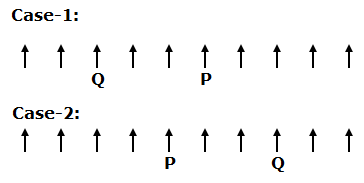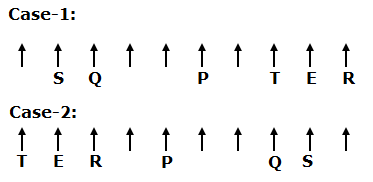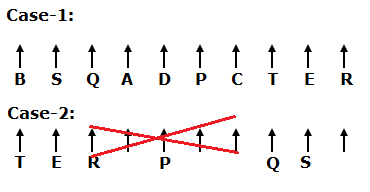# CWC/FCI Prelims 2019 Reasoning Ability Questions (Day-34)

Dear Readers, Exam Race for the Year 2019 has already started, To enrich your preparation here we are providing new series of Practice Questions on CWC/FCI Prelims 2019 Reasoning Ability Questions on daily basis. Aspirants, practice these CWC/FCI Prelims 2019 Reasoning Ability Questions (Day-34) on a regular basis to improve your score in the aptitude section. Start your effective preparation from the right beginning to get success in the upcoming CWC/FCI Exam.

[WpProQuiz 5878]

Direction (1-5): Read the following information carefully and answer the questions given below.

Ten persons A, B, C, D, E, P, Q, R, S and T are sitting in a straight row and all of them are facing north but they are not necessary to besit in the same order.

Only two persons are sitting between P and Q, who sits third from the either ends of the row. E sits immediate right of T and none of them are immediate neighbours of P. As many persons sitting to the right of E is same as sitting to the left of S. S does not sit any of the extreme ends of the row. Only one person sitting between T and R. B sits fourth to the left of D, who does not sit at the extreme end. As many persons sitting between E and P is same as sitting between B and A.

1) Who among the following persons sits third to the right of A?

a) T

b) B

c) S

d) C

e) D

2) How many persons are sitting between C and S?

a) None

b) One

c) Two

d) Three

e) More than three

3) Which of the following pairs sits at the extreme ends of the row?

a) B, E

b) T, R

c) R, B

d) A, Q

e) None of these

4) If P is related to D and B is related to R in a certain way. Then, T is related to which of the following?

a) C

b) Q

c) P

d) S

e) E

5) If all the persons are made to sit as in the alphabetical order from left to right, then how many of them remains the same postion?

a) None

b) One

c) Two

d) Three

e) More than three

Direction (6-10): In these questions, a relationship between different elements is shown in the statements. The statements are followed by two conclusions.

a) If only conclusion I follows.

b) If only conclusion II follows

c) If either conclusion I or II follows

d) If neither conclusion I nor II follows

e) If both conclusion I and II are follows

6) Statements: A≤D<F=H≥M; G=D≤N<I.

Conclusions:

1. F>I
2. M≤N

7) Statements: P≥Q=M≥O<T; N≥V=O≤S.

Conclusions:

1. V≤P
2. Q>V

8) Statements: W=M≤S<E>F=T; J≤K=E<O.

Conclusions:

1. K>W
2. M<O

9) Statements: D<G=K≤M>W>X; M≤E=H<N.

Conclusions:

1. X≤E
2. D<E

10) Statements: G≤B≤N=U>T; R≥T>P=A≥S.

Conclusions:

1. N>A
2. R≥B

Direction (1-5):• Only two persons are sitting between P and Q, who sits third from the either ends of the row.• E sits immediate right of T and none of them are immediate neighbours of P. As many persons sitting to the right of E is same as sitting to the left of S. S does not sit any of the extreme ends of the row. Only one person sitting between T and R.• B sits fourth to the left of D, who does not sit at the extreme end.
• So, Case-2 will be dropped.
• As many persons sitting between E and P is same as sitting between B and A.Direction (6-10) :

1. F>I -> F>D≤N<I
2. M≤N -> M≤H=F>D≤N

1. V≤P -> V=O≤M=Q≤P
2. Q>V -> Q=M≥O=V

1. K>W -> K=E>S≥M=W
2. M<O -> M≤S<E<O

1. X≤E -> X<W<M≤E
2. D<E -> D<G=K≤M≤E